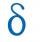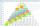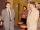# AP 6

Calculate the first five items of an arithmetic sequence if it is given:
a2 – a3 + a5 = 20
a1 + a6 = 38

a =  14
a2 =  16
a3 =  18
a4 =  20
a5 =  22

### Step-by-step explanation:

${a}_{2}=a+d=16$
${a}_{3}={a}_{2}+d=18$
${a}_{4}={a}_{3}+d=20$
${a}_{5}={a}_{4}+d=22$Did you find an error or inaccuracy? Feel free to write us. Thank you!Tips to related online calculators
Do you have a linear equation or system of equations and looking for its solution? Or do you have a quadratic equation?

## Related math problems and questions:

• AP - basicsDetermine first member and differentiate of the the following sequence: a3-a5=24 a4-2a5=61
• SequenceWrite the first 6 members of this sequence: a1 = 5 a2 = 7 an+2 = an+1 +2 an
• SequenceIn the arithmetic sequence is given: Sn=2304, d=2, an=95 Calculate a1 and n.
• Geometric sequenceIn the geometric sequence is a4 = 20 a9 = -160. Calculate the first member a1 and quotient q.
• SequenceWrite the first 7 members of an arithmetic sequence: a1=-3, d=6.
• Difference APCalculate the difference of arithmetic progression if the sum of its first 19 members Sn = 8075 and the first member is a1 = 20
• AP - simpleFind the first ten members of the sequence if a11 = 132, d = 3.
• Geometric sequence 5About members of geometric sequence we know: ? ? Calculate a1 (first member) and q (common ratio or q-coefficient)
• Sequences AP + GPThe three numbers that make up the arithmetic sequence have the sum of 30. If we subtract from the first 5, from the second 4 and keep the third, we get the geometric sequence. Find AP and GP members.
• AP - simpleDetermine the first nine elements of sequence if a10 = -1 and d = 4
• 6 termsFind the first six terms of the sequence. a1 = 7, an = an-1 + 6
• Geometric sequence 3In geometric sequence is a8 = 312500; a11= 39062500; sn=1953124. Calculate the first item a1, quotient q, and n - number of members by their sum s_n.
• Arithmetic progressionIn some AP applies: 5a2 + 7a5 = 90 s3 = 12 Find the first member a =? and difference d = ?
• Geometric sequence 4It is given geometric sequence a3 = 7 and a12 = 3. Calculate s23 (= sum of the first 23 members of the sequence).
• Two workersTwo workers make 138 parts/items. The first one produces 30% more than the second. How many items will each produce?
• Sequence 2Write the first 5 members of an arithmetic sequence a11=-14, d=-1
• SequenceIn the arithmetic sequence is a1=-1, d=4. Which member is equal to the number 203?